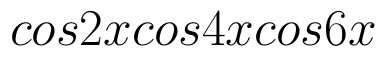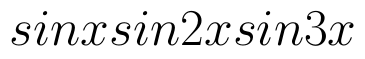Select Page

# CBSE 12 Science Answers for MCQ Maths Application of Derivatives in English

CBSE 12 Science Answers for MCQ Maths Application of Derivatives in English to enable students to get Answers in a narrative video format for the specific question.

Expert Teacher provides CBSE 12 Science Answers for MCQ Maths Application of Derivatives through Video Answers in English language. This video solution will be useful for students to understand how to write an answer in exam in order to score more marks. This teacher uses a narrative style for a question from Application of Derivatives not only to explain the proper method of answering question, but deriving right answer too.

Please find the question below and view the Answer in a narrative video format.

Question:

## Similar Questions from CBSE, 12th Science, Maths, Application of Derivatives

Question 1 : For all values of x, the minimum value ofis : (View Answer Video)

Question 2 : Find two numbers whose sum is 24 and whose product is as large as possible. (View Answer Video)

Question 3 : For all real values of x the minimum value of.(View Answer Video)

Question 4 : The slope of the tangent to the curve:Question 5 : A balloon, which always remains spherical has a variable radius. Find the rate at which its volume is increasing with the radius when the later is 10 cm.  (View Answer Video)

### Inverse Trigonometric Functions

Question 1 : Write the principal value of(View Answer Video)

Question 2 : Evaluate :(View Answer Video)

Question 3 :is equal to : (View Answer Video)

Question 4 : Write the principal value of(View Answer Video)

Question 5 : Write in the simplest form:(View Answer Video)

### Integrals

Question 1 :  Find the integral of the function. (View Answer Video)

Question 2 : Evaluate :(View Answer Video)

Question 3 : Find :(View Answer Video)

Question 4 : Find the integral of the function. (View Answer Video)

Question 5 : Find the integral of the function. (View Answer Video)

### Three Dimensional Geometry

Question 1 : If a line has direction ratios 2, -1, -2, then what are its direction cosines? (View Answer Video)

Question 2 : Write the direction ratio's of the vectorwhereand(View Answer Video)

Question 3 : Find the angle between the linesand(View Answer Video)

Question 4 : Find the distance between the point (7, 2, 4) and the plane determined by the points A(2, 5, -3), B(-2, -3, 5) and C(5, 3, -3). (View Answer Video)

Question 5 : Write the sum of intercepts cut off by the planeon the three axes.  (View Answer Video)